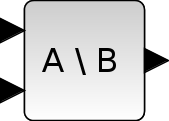Scilab Home page | Wiki | Bug tracker | Forge | Mailing list archives | ATOMS | File exchange
Change language to: English - Français - Português - 日本語 -
Справка Scilab >> Xcos > palettes > Matrix operation palette > MATBKSL

# MATBKSL

Left matrix division

### Block Screenshot### Description

The MATBKSL block outputs the left matrix division. It is a solution to A*x=B. The higher input is the A matrix, the lower one is the B matrix, and the output is x. If A is an MxN1 matrix, B must be a MxN2 matrix where N1 and N2 can be different or equal. The output x is a N1xN2 matrix.

The equivalent of MATBKSL is backslash (\) in Scilab.

### Parameters• Datatype (1=real double 2=Complex)

It indicates the type of the output. It support only the two types double (1) and complex (2). If we input another entry in this label xcos will print the message "Datatype is not supported".

Properties : Type 'vec' of size 1.

### Default properties

• always active: no

• direct-feedthrough: yes

• zero-crossing: no

• mode: no

• regular inputs:

- port 1 : size [-1,-2] / type 1

- port 2 : size [-1,-3] / type 1

• regular outputs:

- port 1 : size [-2,-3] / type 1

• number/sizes of activation inputs: 0

• number/sizes of activation outputs: 0

• continuous-time state: no

• discrete-time state: no

• object discrete-time state: no

• name of computational function: mat_bksl

### Example

```A = [1 7 3;23 32 29]
B = [21 18;13 10]
then the result of the A*x=B equation is (A\B):
x = [-4.504 -3.922;3.643 3.132;0.000 0.000]
```

Example of left division: tan(x) = sin(x)/cos(x)### Interfacing function

• SCI/modules/scicos_blocks/macros/MatrixOp/MATBKSL.sci

### Computational function

• SCI/modules/scicos_blocks/src/c/mat_bksl.c
• SCI/modules/scicos_blocks/src/c/matz_bksl.c